# Graph in Data Structures

Amit Kumar Ghosh  27 min read
21 Sep 2023
281 Views

## Graph in Data Structure: An Overview

Having a strong understanding of data structure and the ability to use proper algorithms, is an essential part of advancing your career in software engineering or even as a hobby. Graphs are one of the most elegant yet simple solutions to represent relationships within data, granting the user greater insight into not only interconnecting pieces of information but also ways in which you can explore it all further. In this article, we'll cover what defines graphs and why they're such a powerful tool for producing insights from complicated datasets.

## What is a graph in Data Structure?

In computer science and data structures, a graph in data structure is a non-linear data structure that consists of a set of vertices (also known as nodes) and a bunch of edges connecting these vertices. It is used to represent relationships or connections between objects. The vertices in a graph can represent various entities such as cities, people, web pages, or any other item of interest, depending on the context. The edges represent the connections or relationships between the vertices. An edge connects two vertices and can be either directed or undirected.

## Types of Graphs in Data Structures

Each of the several graph types found in data structures is described here:

### Finite Graph

If there are a finite number of edges and vertices in the graph G=(V, E), the graph is said to be finite.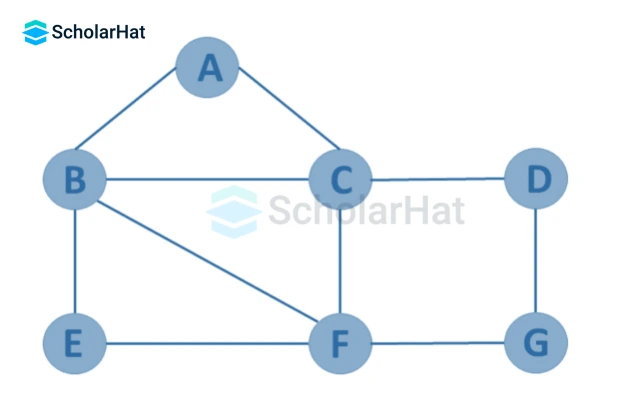### Infinite Graph

If there are an infinite number of edges and vertices in the graph G=(V, E), the graph is said to be infinite.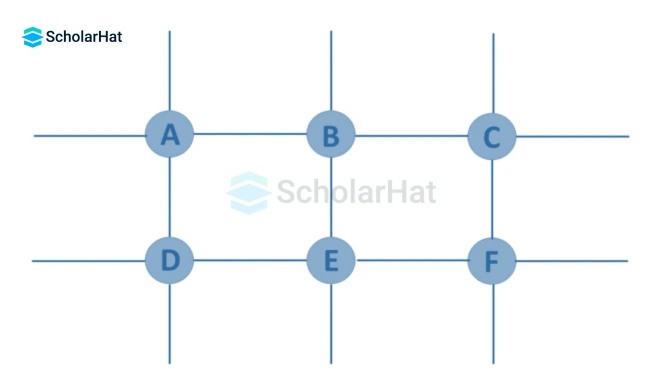### Trivial Graph

If a graph G=(V, E) has just one vertex and no edges, it is referred to as trivial.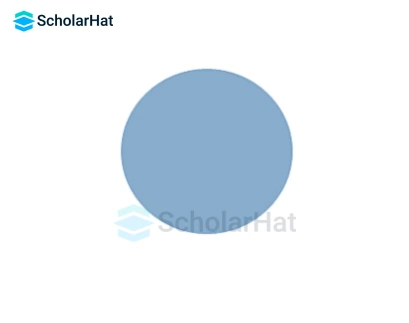### Simple Graph

A graph G=(V, E) is deemed simple if each pair of nodes or vertices contains just one edge. In order to represent one-to-one interactions between two elements, there is only one edge connecting two vertices.### Multi Graph

A graph G=(V, E) is referred to as a multigraph if many edges connect any two vertices. A Multigraph doesn't contain any self-loops.### Null Graph

It's a revised version of a trivial graph. A graph G=(V, E) is a null graph if it has many vertices but none of them are connected by any edges.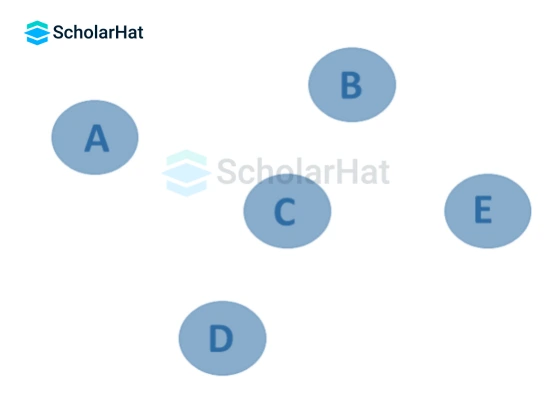### Complete Graph

It is complete if a graph G= (V, E) is also a simple graph. Edges having a n number of vertices must be connected. It is also known as a full graph since the degree of each vertex must be n-1.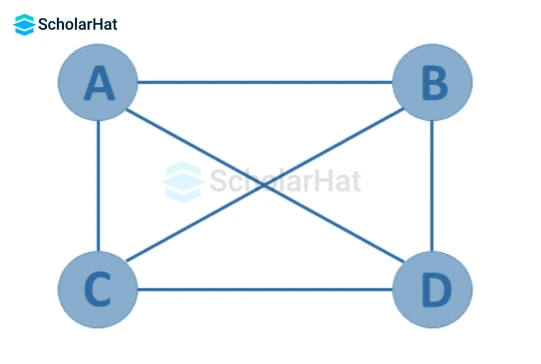### Pseudo Graph

A pseudograph exists when a graph G= (V, E) contains a self-loop in addition to other edges.### Regular Graph

A regular graph is one in which G= (V, E) is a simple graph with the same degree at each vertex. As a result, every graph in its whole is a regular graph.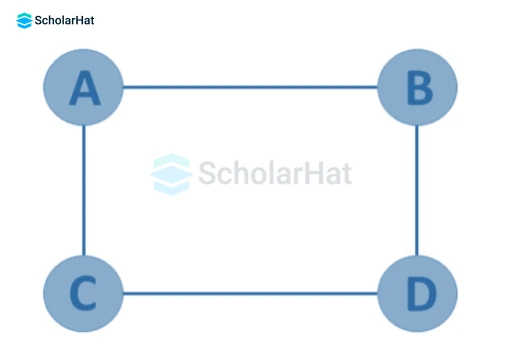### Weighted Graph

A graph G=(V, E) is referred to as a labeled or weighted graph because each edge contains a value or weight that indicates how much it will cost to traverse that edge.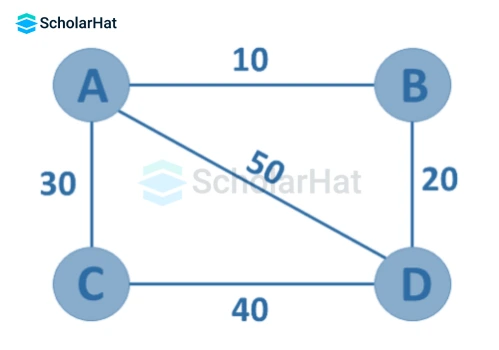### Directed Graph

A directed graph, also known as a digraph, is a collection of nodes connected by edges, each with a distinct direction.### Undirected Graph

An undirected graph is made up of nodes and the links that connect them. The order of the two connected vertices is meaningless and has no bearing. With a finite number of vertices and edges, you can create an undirected graph.### Connected Graph

The graph is connected if there is a path connecting one vertex of a graph data structure to any other vertex.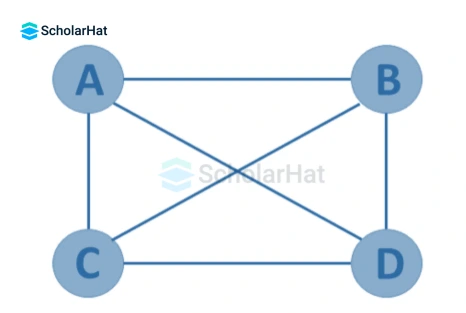### Disconnected Graph

When there is no edge connecting the vertices, the null graph is referred to as a disconnected graph.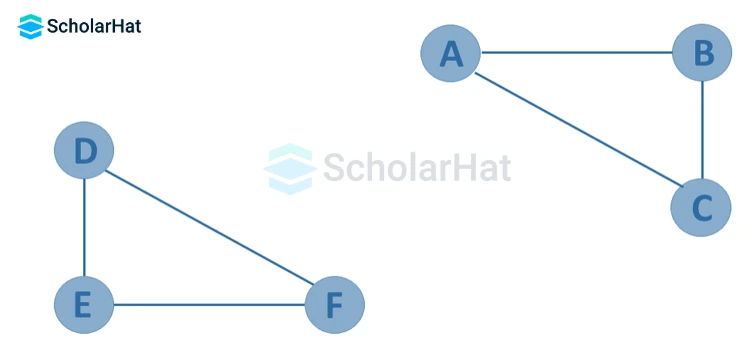### Cyclic Graph

A graph is termed cyclic if it has at least one graph cycle.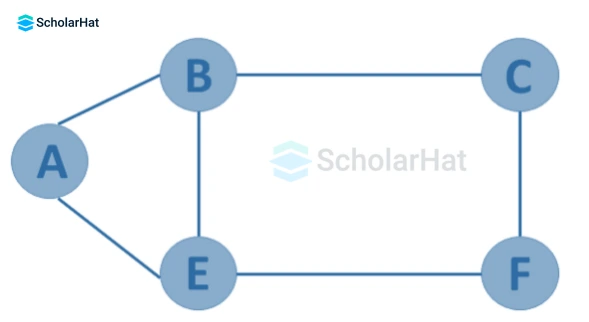### Acyclic Graph

A graph is said to be acyclic if it contains no cycles.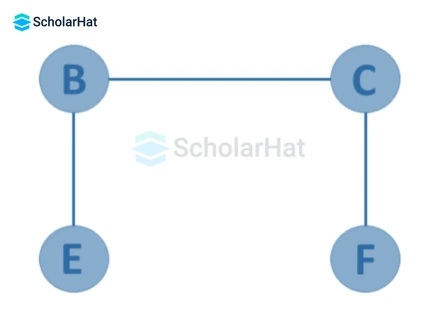### Directed Acyclic Graph

It is a graph with directed edges but no cycle, and it is also known as a directed acyclic graph (DAG). Because it guides the vertices and maintains certain data, it depicts the edges with an ordered pair of vertices.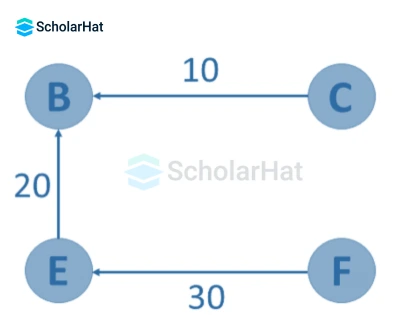### Subgraph

A subgraph is a set of vertices and edges in one graph that are subsets of another.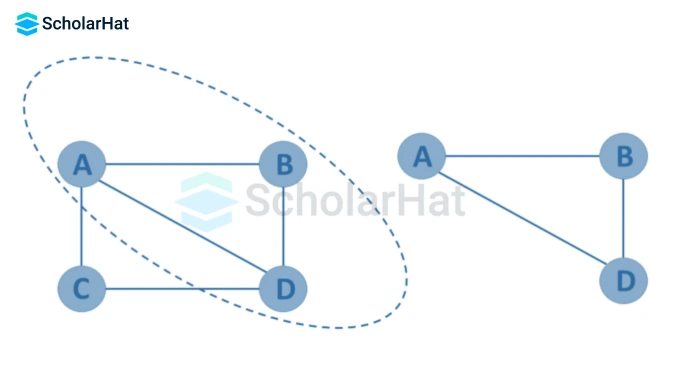## Graph terminology in data structure

Graph terminology in data structure refers to the specific vocabulary and concepts used to describe and analyze graphs, which are mathematical structures composed of nodes (also called vertices) connected by edges. Here are some components of graphs used in graph theory:

1. Node/Vertex: A fundamental unit of a graph. It represents an entity or an element and is usually depicted as a point.
2. Edge/Arc: A connection between two nodes. It represents a relationship or a link between the corresponding entities. An edge can be directed (arc), indicating a one-way connection, or undirected, representing a two-way connection.
3. Adjacent Nodes: Two nodes that are directly connected by an edge. In an undirected graph, both nodes are considered adjacent to each other. In a directed graph, adjacency depends on the direction of the edge.
4. Degree: The degree of a node is the number of edges incident to it, i.e., the number of edges connected to that node. In a directed graph, the degree is divided into two categories: the in-degree (number of incoming edges) and the out-degree (number of outgoing edges).
5. Path: A path in a graph is a sequence of edges that connects a sequence of nodes. It can be a simple path (no repeated nodes) or a closed path/cycle (starts and ends at the same node).
6. Connected Graph: A graph is considered connected if there is a path between every pair of nodes. In other words, there are no isolated nodes or disconnected components.
7. Directed Acyclic Graph (DAG): A directed graph with no directed cycles. This means there is no sequence of directed edges that starts and ends at the same node.
8. Weighted Graph: A graph in which each edge is assigned a numerical value or weight. These weights can represent distances, costs, or any other relevant metric.
9. Bipartite Graph: A graph whose nodes can be divided into two disjoint sets such that every edge connects a node from one set to a node from the other set. In other words, there are no edges between nodes within the same set.
10. Spanning Tree: A subgraph of a connected graph that includes all the nodes of the original graph and forms a tree (a connected acyclic graph) by eliminating some of the edges.
11. Cycle: A closed path in a graph, where the first and last nodes are the same. It consists of at least three edges.

## Graph representation in data structure

• Graph representation is a way of structuring and visualizing data using nodes (vertices) and edges. It is a powerful data structure that is widely used in computer science and various domains to model relationships and connections between different entities.
• In a graph, nodes represent individual entities, while edges represent the relationships or connections between those entities. The connections can be directional or undirected, depending on whether the edges have a specific direction or not. Here are two common types of graphs:

## Adjacency matrix representation of the graph

• An adjacency matrix is a sequential representation.
• It is used to indicate which nodes are adjacent to one another.
• For this representation, you generate an MXM matrix G.
• If there is an edge between vertex a and vertex b, the corresponding element of G, gi,j, equals 1, otherwise gi,j equals 0.
• If the graph is weighted, you can record the weight of the edge instead of 1s and 0s.

### Weighted Undirected Graph Representation

A weighted graph representing these values in the matrix is indicated at the graph's edge.Adjacency list representation of the graph

An adjacency list is a data structure used to represent connections or relationships between elements in a graph. In an adjacency list, each element or vertex in the graph is associated with a list of its neighboring vertices. This representation is commonly used when the graph is sparse, meaning it has relatively few connections compared to the total number of vertices.

### Weighted Undirected Graph Representation Using Linked-List### Weighted Undirected Graph Representation Using an Array## Operations on Graphs in Data Structure

Graph operations refer to the various actions or manipulations performed on a graph data structure. Graphs are mathematical structures composed of nodes (also known as vertices) and edges, which represent relationships between the nodes. The following operations are performed on graphs in data structures:

• Creating graphs
• Insert vertex
• Delete vertex
• Insert edge
• Delete edge

### Creating graphs

There are two methods for creating a graph:

A basic labeled graph's adjacency matrix, sometimes referred to as the connection matrix, is a matrix with rows and columns labeled by graph vertices and a 1 or 0 in position based on whether or not they are adjacent.

An adjacency list, which is a collection of unordered lists, is used to represent a finite graph. Each unordered list in an adjacency list describes the collection of neighbors of a specific vertex in the graph.

### Insert Vertex

A vertex is added when there are already one or more vertices or nodes in the network. This causes the matrix's row and column sizes to increase by one.### Delete Vertex

A vertex can be deleted by selecting it and deleting it from a graph that has already been saved. The matrix returns a removed node if it can be found in the graph after being removed. The matrix returns the node that is not available if a deleted node is not present in the graph.### Insert Edge

A graph may obtain an edge by joining two of the given vertices.### Delete Edge

An edge can be deleted by severing the link between its vertices or nodes.## Graph Traversal Algorithm

Graph traversal is the process of going through or updating each vertex in a graph. Such traversals are classified according to the order in which they visit the vertices. A subset of tree traversal is graph traversal. A graph traversal algorithm can be implemented using one of two methods:

• Depth-first search

• A search method called BFS is used to locate nodes in a graph data structure that satisfy a set of requirements.
• Starting at the graph's root, it examines each node at the current depth level before moving on to the nodes at the next depth level.
• More memory is typically needed, and a queue is needed to keep track of the child nodes that have been encountered but have not yet been inspected.

### Depth-First Search or DFS

• Using DFS, you can locate a node in a graph data structure that satisfies a set of requirements.
• Data structures like trees and graphs are traversed or explored by the depth-first search (DFS) algorithm.
• Starting at the root node, the DFS algorithm investigates each branch as far as it can go before turning around.
• More memory, typically a stack, is needed to keep track of the child nodes that have been encountered but haven't been inspected.

## Application of Graphs in Data Structures

The following are some data structure applications for graphs:

• In computer science, graphs are used to show how computations go.
• On Facebook, users are referred to as "vertices" and "edges" connect them if they are friends. Graph theory is the foundation of Facebook's Friend Suggestion mechanism.
• The Operating System contains a resource allocation graph where each process and resource is shown vertically. The edges between resources and their designated functions or between the request procedure and the desired resource are drawn. If a cycle is created as a result, there will be no progress.
• On the World Wide Web, web pages are referred to as vertices. Let's say there is a connection between pages A and B that can serve as an edge. This application serves as an example of a directed graph.
• Systems for graph transformation use rules to manipulate graphs stored in memory. Graph databases permanently and safely store and query graph-structured data.

## Code Implementation of Graphs in Data Structure

``````
class Graph:
def __init__(self, n):
self.numVertices = n
self.adjList = [[] for _ in range(n)]

def DFS(self, vertex, visited):
visited[vertex] = True
print(vertex, end=" ")

if not visited[v]:
self.DFS(v, visited)

def DFS(self, startVertex):
visited = [False] * self.numVertices
self.DFS(startVertex, visited)

# Create a graph with 5 vertices
graph = Graph(5)

print("Depth-First Traversal (starting from vertex 0): ", end="")
graph.DFS(0)
print()``````
``````
import java.util.ArrayList;
import java.util.List;

class Graph {
private int numVertices; // Number of vertices

// Constructor
public Graph(int n) {
numVertices = n;
for (int i = 0; i < n; i++) {
}
}

// Add an edge to the graph
public void addEdge(int u, int v) {
}

// Depth-First Search traversal
private void DFS(int vertex, boolean[] visited) {
visited[vertex] = true;
System.out.print(vertex + " ");

for (int v : adjList.get(vertex)) {
if (!visited[v]) {
DFS(v, visited);
}
}
}

// DFS traversal starting from a given vertex
public void DFS(int startVertex) {
boolean[] visited = new boolean[numVertices]; // Array to track visited vertices
DFS(startVertex, visited);
}
}

public class Main {
public static void main(String[] args) {
// Create a graph with 5 vertices
Graph graph = new Graph(5);

System.out.print("Depth-First Traversal (starting from vertex 0): ");
graph.DFS(0);
System.out.println();
}
}``````
``````
#include <iostream>
#include <vector>

using namespace std;

// Graph class
class Graph {
private:
int numVertices; // Number of vertices

public:
// Constructor
Graph(int n) {
numVertices = n;
}

// Add an edge to the graph
void addEdge(int u, int v) {
}

// Depth-First Search traversal
void DFS(int vertex, vector<bool>& visited) {
visited[vertex] = true;
cout << vertex << " ";

for (int v : adjList[vertex]) {
if (!visited[v]) {
DFS(v, visited);
}
}
}

// DFS traversal starting from a given vertex
void DFS(int startVertex) {
vector<bool> visited(numVertices, false); // Array to track visited vertices
DFS(startVertex, visited);
}
};

int main() {
// Create a graph with 5 vertices
Graph graph(5);

cout << "Depth-First Traversal (starting from vertex 0): ";
graph.DFS(0);
cout << endl;

return 0;
}``````

In order to represent an undirected graph and carry out depth-first traversal (DFS) on it, this code defines a class called Graph. A graph with five vertices is created, edges are added between them, and a DFS traversal is then carried out, beginning at vertex 0, reporting the visited vertices in the order they were visited.

#### Output

``Depth-First Traversal (starting from vertex 0): 0 1 2 3 4``

## FAQs

### 1. What is the graph and its types in data structure?

A collection of nodes (vertices) joined by edges makes up a graph in a data structure. Graphs can be classified as directed, undirected, weighted, or unweighted.

### 2. What is a graph in data representation?

A graph is a visual or abstract depiction of relationships between items or entities in data representation.

### 3. What is the importance of a graph?

For modeling complicated relationships, such as those seen in social networks, transportation systems, or computer networks, graphs are essential.

### 4. What are the applications of graph data structure?

Social networks, recommendation systems, routing algorithms, & dependency analysis are all examples of applications for graph data structures.

### 5. What are the operations of graph data structure?

Adding and removing vertices and edges, traversing, locating pathways, and analyzing connectivity are common operations on graphs.

### 6. How are graphs useful when interpreting data?

Graphs are helpful for understanding trends and insights in a variety of industries by analyzing and visualizing data with complex relationships.

##### Summary

In summary, we have discussed the uses, graph in data structures examples, graph data structure and algorithms, representing graphs in data structures, and advantages of a graph in data structure. A graph is an effective way to organize data as it allows for flexibility and better scalability. We understand that one must take appropriate measures prior to using a graph due to the complexity it can bring with it. It is also important to consider existing resources and skills when planning out which type of graph structure would work most effectively. Overall, understanding how graphs in data structure work can give you insight into organizing and managing large amounts of data smoothly.

Similar Articles